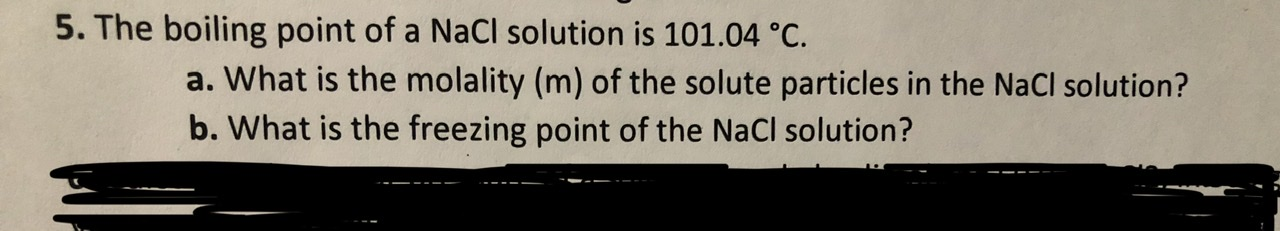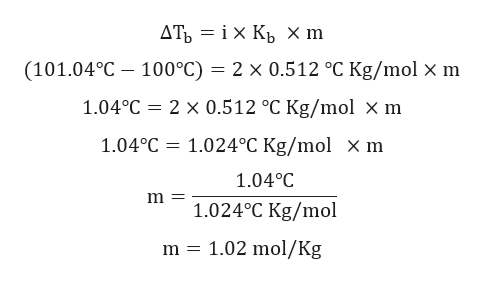# 5. The boiling point of a NaCl solution is 101.04 °C.a. What is the molality (m) of the solute particles in the NaCl solution?b. What is the freezing point of the NaCl solution?

Question
17 viewshelp_outlineImage Transcriptionclose5. The boiling point of a NaCl solution is 101.04 °C. a. What is the molality (m) of the solute particles in the NaCl solution? b. What is the freezing point of the NaCl solution? fullscreen
check_circle

Step 1

Given:

Boiling point of NaCl solution is 100 oC.

Boiling point of pure water is 101.04 oC.

Van't Hoff factor, i will be equal to 2 because we get two moles of particles per mole of sodium chloride added to the solution

The ebullioscopic constant of water is equal to 0.512oC kg mol^−1.

Step 2

Elevation of boiling point is given by:

Step 3

Calculation for molali...help_outlineImage TranscriptioncloseДТЬ — іх Кь xm (101.04°C — 100°C) — 2 х 0.512°С Kg/mol x m 1.04°C 2 x 0.512 °C Kg/mol x m 1.024°C Kg/mol x m 1.04°C 1.04°C 1.024°C Kg/mol 1.02 mol/Kg m = fullscreen

### Want to see the full answer?

See Solution

#### Want to see this answer and more?

Solutions are written by subject experts who are available 24/7. Questions are typically answered within 1 hour.*

See Solution
*Response times may vary by subject and question.
Tagged in

### Solutions